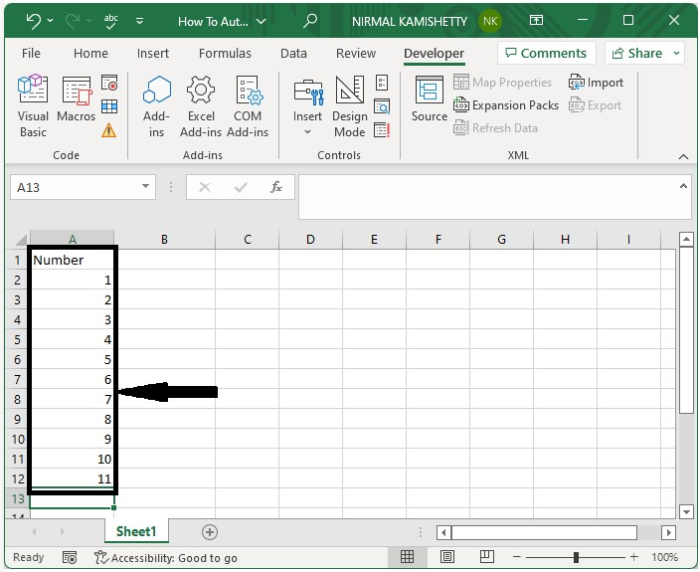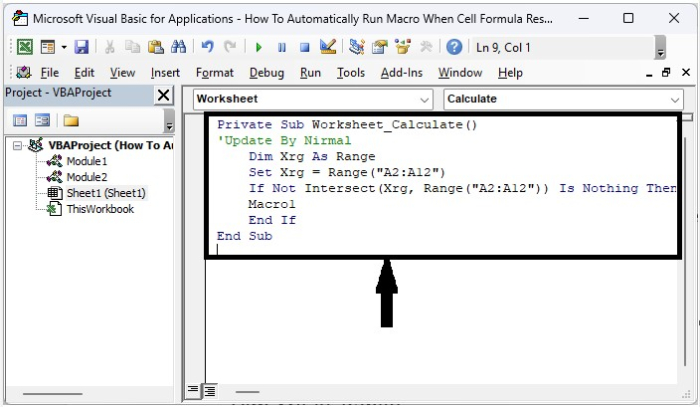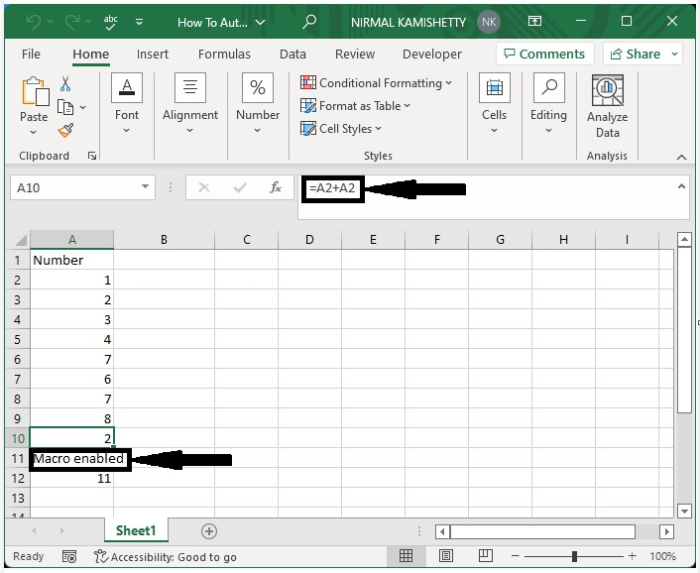# How to Automatically Run a Macro When a Cell Formula Result Changes in Excel?

We need to run a macro by going to the Developer menu and selecting Run Macro or by using the shortcut we created. But have you ever tried to run a macro based on a cell value? It can be done by using the VBA application. In this process, we will automatically run the macro when the value of the specified range has changed. This tutorial will help you understand how we can automatically run macros when the result of a cell formula changes.

Here, we will first select the range of cells and then insert the VBA code for the sheet. Let us see an uncomplicated process to know how we can automatically run macros when cell formula results change in Excel. We need to use the help of a VBA application to complete our process, as we can’t do it by default in Excel.

Step 1

Consider an Excel sheet with data similar to the data shown in the image below, as well as a macro.Now right-click on the sheet name and select View Code to open the VBA application, then type the programme into the text box as shown in the below image.

### Example

Private Sub Worksheet_Calculate()
'Update By Nirmal
Dim Xrg As Range
Set Xrg = Range("A2:A12")
If Not Intersect(Xrg, Range("A2:A12")) Is Nothing Then
Macro1
End If
End Sub


In the code, A2:A12 is the range of cells we formula on, and Macro1 is the name of the macro that will be run automatically.Step 2

Then save the sheet as a macro-enabled workbook and exit the VBA application by pressing "Alt + Q", followed by closing the sheet. When we reopen the sheet, click on enable code, and every time we change the formula in the range, the macro will run automatically.Note − The macro will only run if there is a change in the formula; it will not run if there is a change in any data.

## Conclusion

In this tutorial, we used a simple example to demonstrate how we can automatically run macros when cell formula results change in Excel.